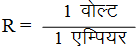# Physics

### Chapter : 1. Electricity

#### Ohm's Law

Ohm's Law
Definition : According to the Ohm’s law at constant temperature, the current flowing through a conductor is directly proportional to the potential difference across the conductor.
Thus, if I is the current flowing through a conductor and V is the potential difference (or voltage) across the conductor, then according to Ohm’s law.
I ∝ V (when T is constant)
or, I = V/R ...(i)
where R is a constant called the resistance of the conductor.
Equation (i) may be written as, -
V = I x R ...(ii)
Unit of resistance :
The SI unit of resistance (R) is ohm. Ohm is denoted by the Greek letter omega (Ω).
From Ohm’s law, R = V/I
Now, if, V = 1 Volt and I = 1 Ampere
Then,Thus, 1 ohm is defined as the resistance of a conductor which allows a current of 1 ampere to flow through it when a potential difference of 1 volt is maintained across it.
Results of Ohm’s Law
Current flowing through a conductor is directly proportional to the potential difference across the conductor.When the potential difference in a circuit is kept constant, the current in inversely proportional to the resistance of the conductor.
I ∝ 1/R
The ratio of potential difference to the current is constant. The value of the constant is equal to the resistance of the conductor (or resistor).
V/I = R